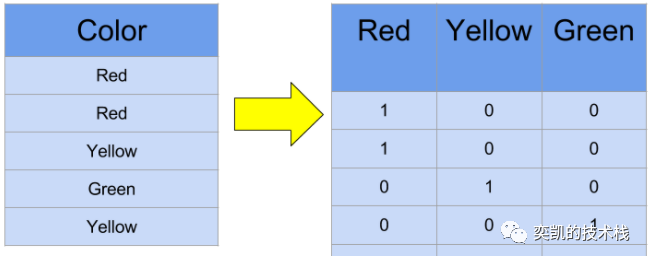0

# Spark进行独热编码

Pandas 进行独热编码是非常简单的，那么如果使用 Spark该怎么做Spark 非常擅长处理 PB 级的数据。但在复杂的建模技术方面，Spark 的 对于ML 支持还不够强大。所以是很多时候数据预处理是在 Spark 中完成，而下游建模是用纯 Python 或其他高级语言（如 Julia）实现的。

``````from pyspark.sql import SparkSession
spark = SparkSession.builder \
.appName("One_Hot_Encoding_Blog") \
.getOrCreate()
.csv("ohe_explanation.csv")
df.show()
``````

``````+------+
| Color|
+------+
|   Red|
|   Red|
|Yellow|
| Green|
|Yellow|
+------+
``````

``````from pyspark.ml.feature import StringIndexer
from pyspark.ml.feature import OneHotEncoder

indexer = StringIndexer(inputCol="Color", outputCol="ColorNumericIndex")
df = indexer.fit(df).transform(df)
ohe = OneHotEncoder(inputCol="ColorNumericIndex", outputCol="ColorOHEVector")
df = ohe.fit(df).transform(df)
df.show()
``````

``````+------+-----------------+--------------+
| Color|ColorNumericIndex|ColorOHEVector|
+------+-----------------+--------------+
|   Red|              0.0| (2,,[1.0])|
|   Red|              0.0| (2,,[1.0])|
|Yellow|              1.0| (2,,[1.0])|
| Green|              2.0|     (2,[],[])|
|Yellow|              1.0| (2,,[1.0])|
+------+-----------------+--------------+
``````

``````df.write.option("header", True).csv("ohe_result.csv")
``````

``````pyspark.sql.utils.AnalysisException: CSV data source does not support struct<type:tinyint,size:int,indices:array<int>,values:array<double>> data type.
``````

``````from pyspark.sql.functions import udf, col
from pyspark.sql.types import IntegerType
``````

1. 收集列中需要进行编码的所有不同值
2. 为每个收集的值创建一个新列，其列名格式为 <<original column name>>_ <<distinct value>>，表示记录中存在 (1) 或不存在 (0)
``````distinct_values = list(df.select("Color")
.distinct()
.toPandas()["Color"])
``````

``````ImportError: Pandas >= 0.23.2 must be installed; however, it was not found.
``````

Pandas能够帮助我们处理很多事情，但实际上可能存在各种限制，例如无法在执行环境中安装新库。在这样的情况下，我们可以使用一个更 Spark 原生的方式，它不需要安装任何额外的库。

``````distinct_values = df.select("Color")\
.distinct()\
.rdd\
.flatMap(lambda x: x).collect()
``````

``````for distinct_value in distinct_values:
function = udf(lambda item:
1 if item == distinct_value else 0,
IntegerType())
new_column_name = "Color"+'_'+distinct_value
df = df.withColumn(new_column_name, function(col("Color")))
df.show()
``````

``````+------+-----------+------------+---------+
| Color|Color_Green|Color_Yellow|Color_Red|
+------+-----------+------------+---------+
|   Red|          0|           0|        1|
|   Red|          0|           0|        1|
|Yellow|          0|           1|        0|
| Green|          1|           0|        0|
|Yellow|          0|           1|        0|
+------+-----------+------------+---------+
``````

### “Spark进行独热编码”的评论:

##### 关于作者##### 奕凯